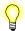# atan(), atanf(), atanl()

Compute the arctangent of an angle

## Synopsis:

```#include <math.h>

double atan( double x );

float atanf( float x );

long double atanl( long double x );```

## Arguments:

x
The tangent for which you want to find the angle.

## Library:

libm

Use the -l m option to qcc to link against this library.

## Description:

These functions compute the arctangent (in radians) of x.

## Returns:

The arctangent, in the range (-π/2, π/2). For finite values of |x| > 1, these functions return NaN. The return value for +/-Inf is NaN.If an error occurs, these functions return 0, but this is also a valid mathematical result. If you want to check for errors, set errno to 0, call the function, and then check errno again. These functions don't change errno if no errors occurred.

## Examples:

```#include <stdio.h>
#include <math.h>
#include <stdlib.h>

int main( void )
{
printf( "%f\n", atan(.5) );
return EXIT_SUCCESS;
}```

produces the output:

`0.463648`

## Classification:

Safety:
Cancellation point No
Interrupt handler No
Signal handler No